# Review Python

10 de Jun de 2020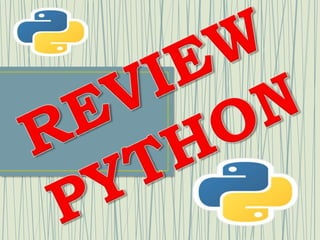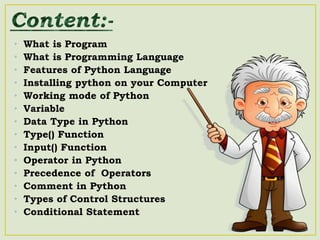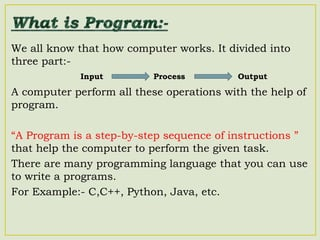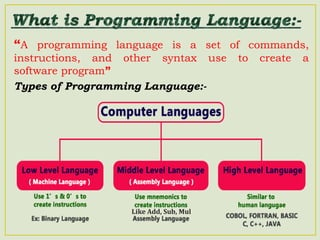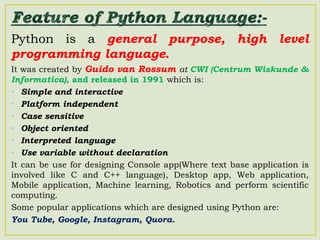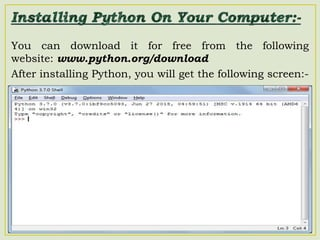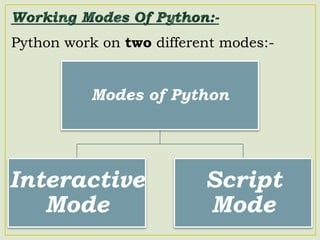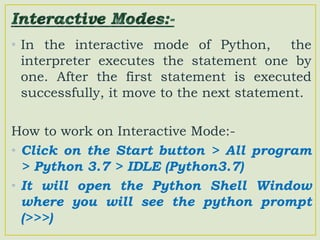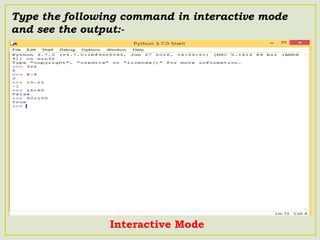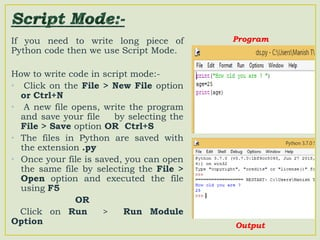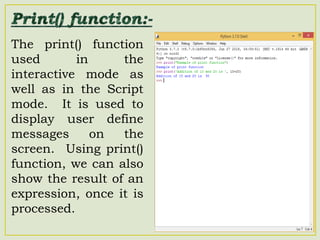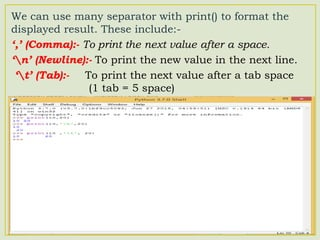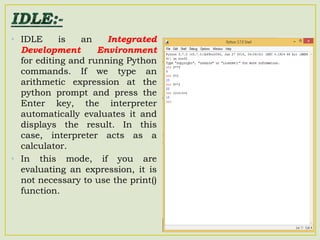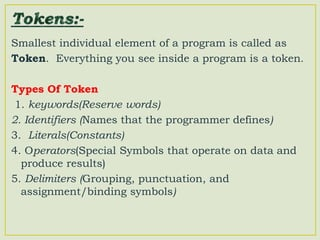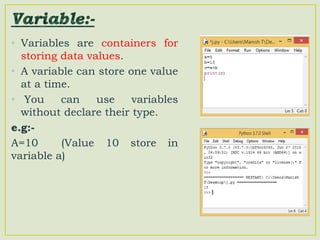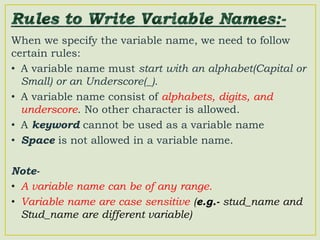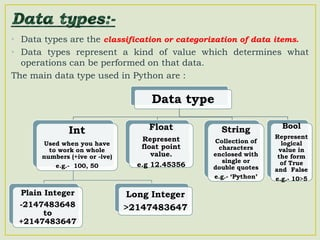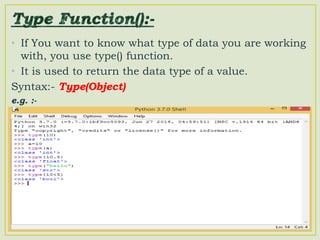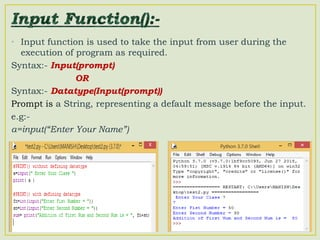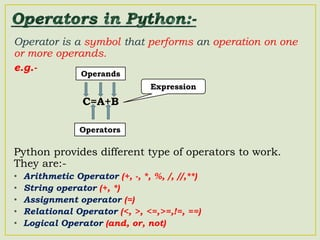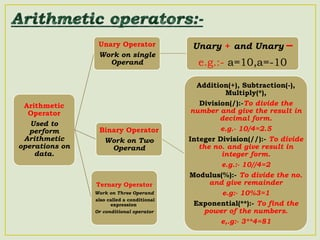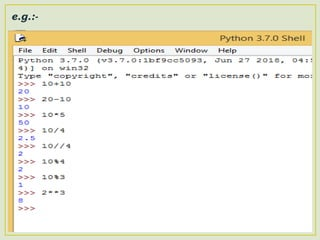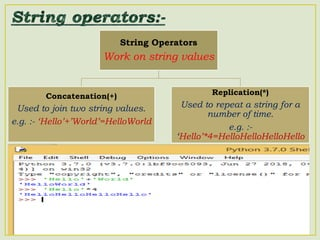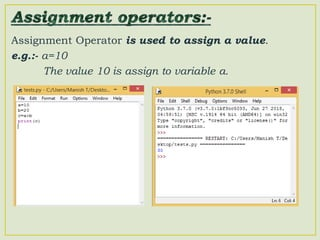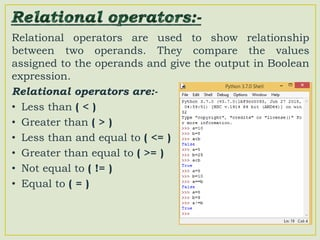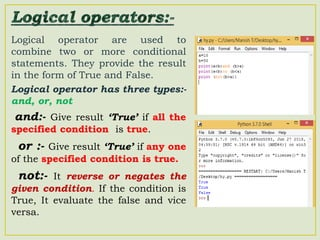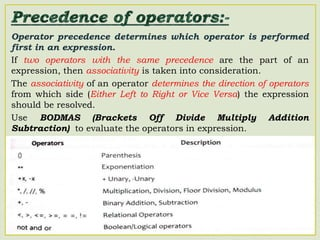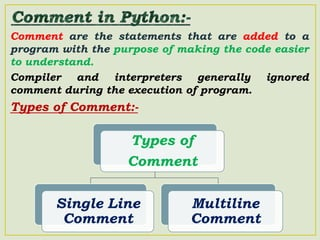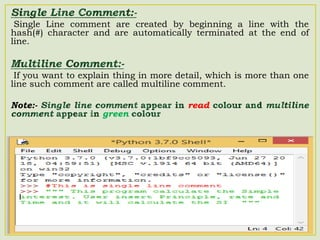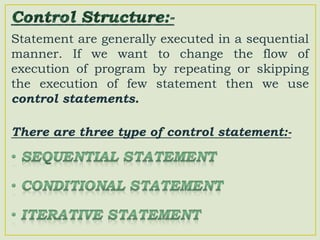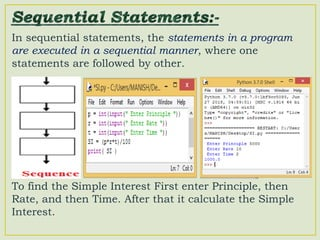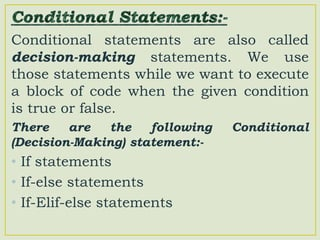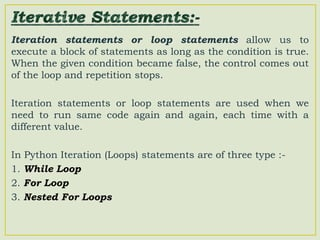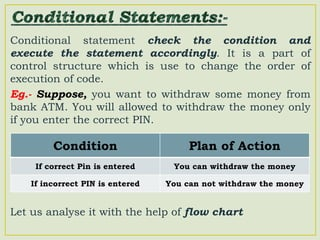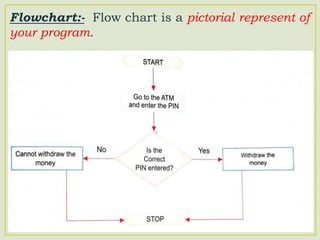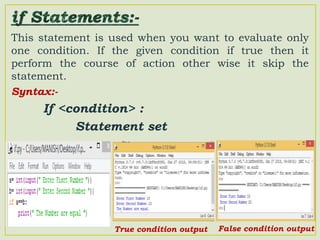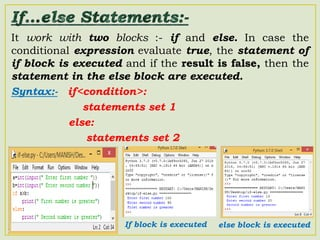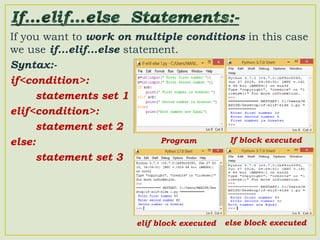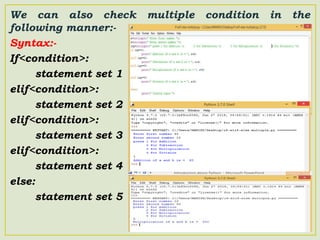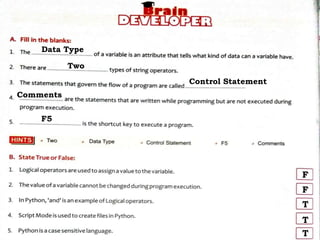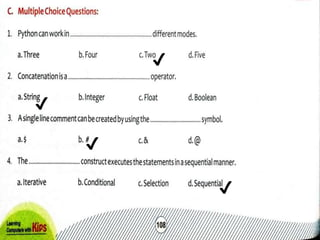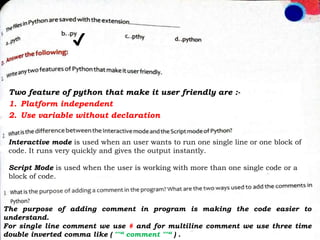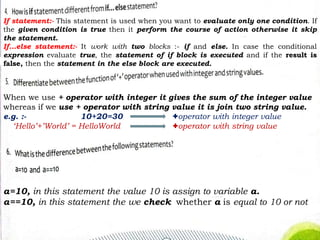1 de 43

### Review Python

• 2. • What is Program • What is Programming Language • Features of Python Language • Installing python on your Computer • Working mode of Python • Variable • Data Type in Python • Type() Function • Input() Function • Operator in Python • Precedence of Operators • Comment in Python • Types of Control Structures • Conditional Statement
• 3. We all know that how computer works. It divided into three part:- A computer perform all these operations with the help of program. “A Program is a step-by-step sequence of instructions ” that help the computer to perform the given task. There are many programming language that you can use to write a programs. For Example:- C,C++, Python, Java, etc. Input Process Output
• 4. “A programming language is a set of commands, instructions, and other syntax use to create a software program” Types of Programming Language:- Like Add, Sub, Mul
• 5. Python is a general purpose, high level programming language. It was created by Guido van Rossum at CWI (Centrum Wiskunde & Informatica), and released in 1991 which is: • Simple and interactive • Platform independent • Case sensitive • Object oriented • Interpreted language • Use variable without declaration It can be use for designing Console app(Where text base application is involved like C and C++ language), Desktop app, Web application, Mobile application, Machine learning, Robotics and perform scientific computing. Some popular applications which are designed using Python are: You Tube, Google, Instagram, Quora.
• 7. Python work on two different modes:- Modes of Python Interactive Mode Script Mode
• 8. • In the interactive mode of Python, the interpreter executes the statement one by one. After the first statement is executed successfully, it move to the next statement. How to work on Interactive Mode:- • Click on the Start button > All program > Python 3.7 > IDLE (Python3.7) • It will open the Python Shell Window where you will see the python prompt (>>>)
• 9. Type the following command in interactive mode and see the output:- Interactive Mode
• 10. If you need to write long piece of Python code then we use Script Mode. How to write code in script mode:- • Click on the File > New File option or Ctrl+N • A new file opens, write the program and save your file by selecting the File > Save option OR Ctrl+S • The files in Python are saved with the extension .py • Once your file is saved, you can open the same file by selecting the File > Open option and executed the file using F5 OR Click on Run > Run Module Option Output Program
• 11. The print() function used in the interactive mode as well as in the Script mode. It is used to display user define messages on the screen. Using print() function, we can also show the result of an expression, once it is processed.
• 12. We can use many separator with print() to format the displayed result. These include:- ‘,’ (Comma):- To print the next value after a space. ‘n’ (Newline):- To print the new value in the next line. ‘t’ (Tab):- To print the next value after a tab space (1 tab = 5 space)
• 13. • IDLE is an Integrated Development Environment for editing and running Python commands. If we type an arithmetic expression at the python prompt and press the Enter key, the interpreter automatically evaluates it and displays the result. In this case, interpreter acts as a calculator. • In this mode, if you are evaluating an expression, it is not necessary to use the print() function.
• 14. Smallest individual element of a program is called as Token. Everything you see inside a program is a token. Types Of Token 1. keywords(Reserve words) 2. Identifiers (Names that the programmer defines) 3. Literals(Constants) 4. Operators(Special Symbols that operate on data and produce results) 5. Delimiters (Grouping, punctuation, and assignment/binding symbols)
• 15. • Variables are containers for storing data values. • A variable can store one value at a time. • You can use variables without declare their type. e.g:- A=10 (Value 10 store in variable a)
• 16. When we specify the variable name, we need to follow certain rules: • A variable name must start with an alphabet(Capital or Small) or an Underscore(_). • A variable name consist of alphabets, digits, and underscore. No other character is allowed. • A keyword cannot be used as a variable name • Space is not allowed in a variable name. Note- • A variable name can be of any range. • Variable name are case sensitive (e.g.- stud_name and Stud_name are different variable)
• 17. • Data types are the classification or categorization of data items. • Data types represent a kind of value which determines what operations can be performed on that data. The main data type used in Python are : Data type Int Used when you have to work on whole numbers (+ive or -ive) e.g.- 100, 50 Plain Integer -2147483648 to +2147483647 Long Integer >2147483647 Float Represent float point value. e.g 12.45356 String Collection of characters enclosed with single or double quotes e.g.- ‘Python’ Bool Represent logical value in the form of True and False e.g.- 10>5
• 18. • If You want to know what type of data you are working with, you use type() function. • It is used to return the data type of a value. Syntax:- Type(Object) e.g. :-
• 19. • Input function is used to take the input from user during the execution of program as required. Syntax:- Input(prompt) OR Syntax:- Datatype(Input(prompt)) Prompt is a String, representing a default message before the input. e.g:- a=input(“Enter Your Name”)
• 20. Operator is a symbol that performs an operation on one or more operands. e.g.- Python provides different type of operators to work. They are:- • Arithmetic Operator (+, -, *, %, /, //,**) • String operator (+, *) • Assignment operator (=) • Relational Operator (<, >, <=,>=,!=, ==) • Logical Operator (and, or, not) Operators Operands Expression C=A+B
• 21. Arithmetic Operator Used to perform Arithmetic operations on data. Unary Operator Work on single Operand Unary + and Unary – e.g.:- a=10,a=-10 Binary Operator Work on Two Operand Addition(+), Subtraction(-), Multiply(*), Division(/):-To divide the number and give the result in decimal form. e.g.- 10/4=2.5 Integer Division(//):- To divide the no. and give result in integer form. e.g.:- 10//4=2 Modulus(%):- To divide the no. and give remainder e.g:- 10%3=1 Exponential(**):- To find the power of the numbers. e,.g:- 3**4=81 Ternary Operator Work on Three Operand also called a conditional expression. Or conditional operator
• 22. e.g.:-
• 23. String Operators Work on string values Concatenation(+) Used to join two string values. e.g. :- ‘Hello’+’World’=HelloWorld Replication(*) Used to repeat a string for a number of time. e.g. :- ‘Hello’*4=HelloHelloHelloHello
• 24. Assignment Operator is used to assign a value. e.g.:- a=10 The value 10 is assign to variable a.
• 25. Relational operators are used to show relationship between two operands. They compare the values assigned to the operands and give the output in Boolean expression. Relational operators are:- • Less than ( < ) • Greater than ( > ) • Less than and equal to ( <= ) • Greater than equal to ( >= ) • Not equal to ( != ) • Equal to ( = )
• 26. Logical operator are used to combine two or more conditional statements. They provide the result in the form of True and False. Logical operator has three types:- and, or, not and:- Give result ‘True’ if all the specified condition is true. or :- Give result ‘True’ if any one of the specified condition is true. not:- It reverse or negates the given condition. If the condition is True, It evaluate the false and vice versa.
• 27. Operator precedence determines which operator is performed first in an expression. If two operators with the same precedence are the part of an expression, then associativity is taken into consideration. The associativity of an operator determines the direction of operators from which side (Either Left to Right or Vice Versa) the expression should be resolved. Use BODMAS (Brackets Off Divide Multiply Addition Subtraction) to evaluate the operators in expression.
• 28. Comment are the statements that are added to a program with the purpose of making the code easier to understand. Compiler and interpreters generally ignored comment during the execution of program. Types of Comment:- Types of Comment Single Line Comment Multiline Comment
• 29. Single Line comment are created by beginning a line with the hash(#) character and are automatically terminated at the end of line. If you want to explain thing in more detail, which is more than one line such comment are called multiline comment. Note:- read green
• 30. Statement are generally executed in a sequential manner. If we want to change the flow of execution of program by repeating or skipping the execution of few statement then we use control statements. There are three type of control statement:-
• 31. In sequential statements, the statements in a program are executed in a sequential manner, where one statements are followed by other. To find the Simple Interest First enter Principle, then Rate, and then Time. After that it calculate the Simple Interest.
• 32. Conditional statements are also called decision-making statements. We use those statements while we want to execute a block of code when the given condition is true or false. There are the following Conditional (Decision-Making) statement:- • If statements • If-else statements • If-Elif-else statements
• 33. Iteration statements or loop statements allow us to execute a block of statements as long as the condition is true. When the given condition became false, the control comes out of the loop and repetition stops. Iteration statements or loop statements are used when we need to run same code again and again, each time with a different value. In Python Iteration (Loops) statements are of three type :- 1. While Loop 2. For Loop 3. Nested For Loops
• 34. Conditional statement check the condition and execute the statement accordingly. It is a part of control structure which is use to change the order of execution of code. Eg.- Suppose, you want to withdraw some money from bank ATM. You will allowed to withdraw the money only if you enter the correct PIN. Let us analyse it with the help of flow chart Condition Plan of Action If correct Pin is entered You can withdraw the money If incorrect PIN is entered You can not withdraw the money
• 35. Flowchart:- Flow chart is a pictorial represent of your program.
• 36. This statement is used when you want to evaluate only one condition. If the given condition if true then it perform the course of action other wise it skip the statement. Syntax:- If <condition> : Statement set True condition output False condition output
• 37. It work with two blocks :- if and else. In case the conditional expression evaluate true, the statement of if block is executed and if the result is false, then the statement in the else block are executed. Syntax:- if<condition>: statements set 1 else: statements set 2 If block is executed else block is executed
• 38. If you want to work on multiple conditions in this case we use if…elif…else statement. Syntax:- if<condition>: statements set 1 elif<condition>: statement set 2 else: statement set 3 Program If block executed elif block executed else block executed
• 39. We can also check multiple condition in the following manner:- Syntax:- If<condition>: statement set 1 elif<condition>: statement set 2 elif<condition>: statement set 3 elif<condition>: statement set 4 else: statement set 5
• 40. Data Type Two Control Statement Comments F5 F F T T T
• 41. ✔ ✔ ✔ ✔
• 42. Interactive mode is used when an user wants to run one single line or one block of code. It runs very quickly and gives the output instantly. Script Mode is used when the user is working with more than one single code or a block of code. ✔ Two feature of python that make it user friendly are :- 1. Platform independent 2. Use variable without declaration The purpose of adding comment in program is making the code easier to understand. For single line comment we use # and for multiline comment we use three time double inverted comma like ( ""“ comment ""“ ) .
• 43. If statement:- This statement is used when you want to evaluate only one condition. If the given condition is true then it perform the course of action otherwise it skip the statement. If…else statement:- It work with two blocks :- if and else. In case the conditional expression evaluate true, the statement of if block is executed and if the result is false, then the statement in the else block are executed. When we use + operator with integer it gives the sum of the integer value whereas if we use + operator with string value it is join two string value. e.g. :- 10+20=30 +operator with integer value ‘Hello’+’World’ = HelloWorld +operator with string value a=10, in this statement the value 10 is assign to variable a. a==10, in this statement the we check whether a is equal to 10 or not